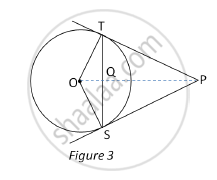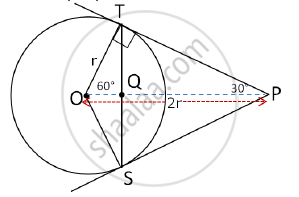# In Fig.3, from an external point P, two tangents PT and PS are drawn to a circle with centre O and radius r. If OP = 2r, show that ∠ OTS = ∠ OST = 30°. - Mathematics

In Fig.3, from an external point P, two tangents PT and PS are drawn to a circle with centre O and radius r. If OP = 2r, show that ∠ OTS = ∠ OST = 30°.#### Solution

In the given figure,

OP = 2r … (Given)

∠OTP = 90° … (radius drawn at the point of contact is perpendicular to the tangent)

In OTP,

sin OPT =(OT)/(OP)=1/2= sin30°

⇒ ∠OPT=30°

∴ ∠TOP=60°

∴ ΔOTP is a 30°-60°-90°, right triangle.In ΔOTS,

OT = OS … (Radii of the same circle)

∴ ΔOTS is an isosceles triangle.

∴ ∠OTS = ∠OST … (Angles opposite to equal sides of an isosceles triangle are equal)

In ΔOTQ and ΔOSQ

OS = OT … (Radii of the same circle)

OQ = OQ ...(side common to both triangles)

∠OTQ = ∠OSQ … (angles opposite to equal sides of an isosceles triangle are equal)

∴ ΔOTQ ≅ ΔOSQ … (By S.A.S)

∴ ∠TOQ = ∠SOQ = 60° … (C.A.C.T)

∴ ∠TOS = 120° … (∠TOS = ∠TOQ + ∠SOQ = 60° + 60° = 120°)

∴ ∠OTS + ∠OST = 180° – 120° = 60°

∴ ∠OTS = ∠OST = 60° ÷ 2 = 30°

Concept: Number of Tangents from a Point on a Circle
Is there an error in this question or solution?
2015-2016 (March) All India Set 1

Share